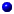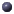In file expr.hpp:

## template<class T> class mat_traits

Trait to access small matrix dimensions

## Documentation

Trait to access small matrix dimensions

### Public Memberstypedef typename T::TELEM TELEM
matrix elementtypedef typename T::TSCAL TSCAL
field of matrix elementtypedef typename T::TV_COL TV_COL
type of column vectortypedef typename T::TV_ROW TV_ROW
type of row vectorenum matrix withtypedef typename T::TELEM TELEM
matrix elementtypedef typename T::TSCAL TSCAL
field of matrix elementtypedef typename T::TV_COL TV_COL
type of column vectortypedef typename T::TV_ROW TV_ROW
type of row vectorenum matrix with
matrix with

This class has no child classes.

This page was generated with the help of DOC++.